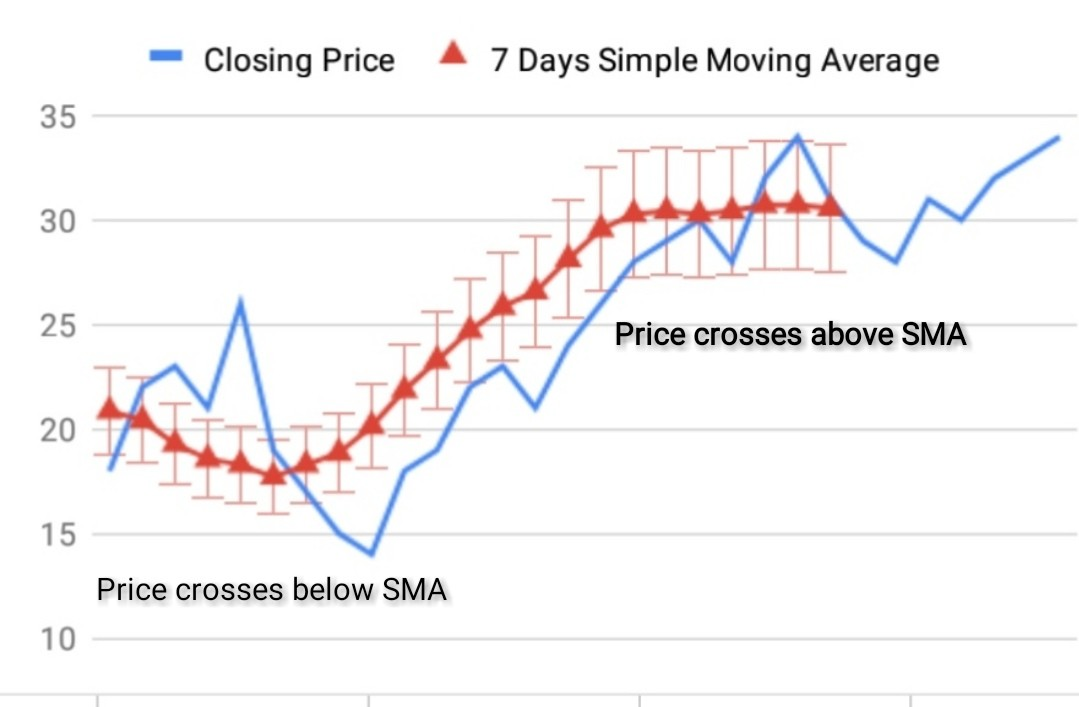What is Simple Moving Average?Learn SMA formula-ArthikDisha

# What is Simple Moving Average?Learn SMA formula# What is Simple Moving Average?Simple Moving Average formula

Since it has been my continuous endeavour to spread investment awareness among the investors, it has also become my responsibility to bring forth the different ideas or philosophies for the mutual fund or stock market investments. Having regard to that today I have decided to write a blog post on What is Simple Moving Average?

Moving Average can be defined as the average recent closing prices of securities over a period of time. It is calculated by totalling the closing prices of securities for a specific period and by dividing it with the period of time. Moving average is one of the most commonly used tools for technical analysis.

Moving average is termed as the lagging indicator of an established trend. Moving Average is basically used to indicate and analyse the price trend of an asset over a given period of time. Moving Average works as a tool to indicate the market or price trend. One of the most important features of the moving average is that it does not predict the market/price direction rather it exhibits the established trend with a lag.

Therefore, it can be said that moving average helps an investor to identify the underlying asset’s momentum and assessing the price level where it would get support or resistance. Investors use the moving average as a tool for deciding the price level at which the underlying asset should be transacted i.e. when to Buy or Sell. Some experts even say that Trend is our best friend.

## What is Simple Moving Average? Simple Moving Average formula

There are basically two moving average tools used by traders to understand and analyse the market trend. In which direction the price is going and the price level where there is a support or resistance. The two moving averages are Simple Moving Average(SMA) and Exponential Moving Average(EMA). But today I will discuss only Simple moving average and the latter I will discuss on later.

## Simple Moving Average

Simple Moving Average or SMA is calculated by taking the average closing prices of securities over a given period of time. However, it can also be computed by taking the day’s open, high and low prices of securities.

#### What we do using simple moving average

Basically, SMA is used as a tool for identifying the price trend but it is mostly used to get the signal for a Buy or Sell decision.  Whether the price direction is also in line with the market trend and the SMA price of the security is showing a Support or Resistance. To make this simple let’s take an example for easy understanding.

Example:   The closing prices of Stock  for the last 7 days is 18,22,23,21,26,19,17.

Now the SMA price is (18+22+23+21+26+19+17)/7 =Rs.20.85.

Let’s now see this in graphical format in a very simple way.

So, we can see from the above chart that the red line is SMA and its value stands at 20.85.The average is called moving because it is plotted on the chart bar by forming a line that moves along the chart as the average value changes. This method is very simple as only the average price is to be calculated over a specific period of time yet useful.

If SMA is moving up it means then the trend is up and similarly, when SMA is down, the trend is also down.

#### How to draw a Simple Moving Average Line and what does it indicate

Let’s take another example to show 7 days simple moving average for Stock X.

 Day Closing Price Average Closing Price 1 18 0 2 22 0 3 23 0 4 21 0 5 26 0 6 19 0 7 17 0 8 15 20.86 7 Days Average Closing Price 9 14 20.43 10 18 19.29 11 19 18.57 12 22 18.29 13 23 17.71 14 21 18.29 15 24 18.86 16 26 20.14 17 28 21.86 18 29 23.29 19 30 24.71 20 28 25.86 21 32 26.57 22 34 28.14 23 31 29.57 24 29 30.29 25 28 30.43 26 31 30.29 27 30 30.43 28 32 30.71 29 33 30.71 30 34 30.57

In the above graph I have used 7 Days SMA. Generally shorter period SMAs are used to determine the shorter period trend and vice versa. Primarily a 200-period SMAs are used to identify the longer period trend and a 50-period SMAs are used to get an intermediate trend.In the above picture, we can see that price crossing both below SMA and above SMA. Price crossing below SMA signals that you need to take a Short Sell position.  On the other hand when price crosses above SMA, this signals that you need to take a Long Buy position.

Now from the below graph you can see the two SMAs altogether. One is 7 Days SMA and the other one is 15 Days SMA.

When the shorter period SMA(7 Days SMA) crosses below the longer period SMA(15 Days SMA), it signals that you need to take a Short  position. Similarly when the shorter period SMA(7 Days SMA) crosses above the longer period SMA(15 Days SMA), it signals that you need to take a Long position.

#### Formula of Simple Moving Average

Basically, simple moving average is the average or mean value of stock price over a specific period of time.